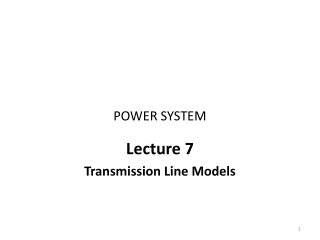DownloadDownload PresentationPOWER SYSTEM

# POWER SYSTEM

Download Presentation## POWER SYSTEM

- - - - - - - - - - - - - - - - - - - - - - - - - - - E N D - - - - - - - - - - - - - - - - - - - - - - - - - - -
##### Presentation Transcript

1. POWER SYSTEM Lecture 7 Transmission Line Models

2. Transmission Line Models • Previous lectures have covered how to calculate the distributed series inductance, shunt capacitance, and series resistance of transmission lines: • That is, we have calculated the inductance L, capacitance C, and resistance r per unit length, • We can also think of the shunt conductance g per unit length, • Each infinitesimal length dx of transmission line consists of a series impedance rdx + jωLdx and a shunt admittance gdx + jωCdx, • In this section we will use these distributed parameters to develop the transmission line models used in power system analysis.

3. dx dx dx Transmission Line Equivalent Circuit • Our model of an infinitesimal length of transmission line is shown below: L Units on z and y are per unit length!

4. dx dx dx Derivation of V, I Relationships L

5. Setting up a Second Order Equation

6. V, I Relationships, cont’d

7. Equation for Voltage

8. Real Hyperbolic Functions

9. Complex Hyperbolic Functions

10. Determining Line Voltage

11. Determining Line Voltage, cont’d

12. Determining Line Current

13. Transmission Line Example

14. Transmission Line Example, cont’d

15. Transmission Line Example, cont’d Squares and crosses show real and reactive power flow, where a positive value of flow means flow to the left. Receiving end Sending end

16. Lossless Transmission Lines

17. Lossless Transmission Lines

18. Lossless Transmission Lines If load power P > SIL then line consumes VArs; otherwise, the line generates VArs.

19. IS IR + + Transmission Line VS VR - - Transmission Matrix Model • Often we are only interested in the terminal characteristics of the transmission line. Therefore we can model it as a “black box:”

20. Transmission Matrix Model, cont’d

21. Equivalent Circuit Model To do this, we’ll use the T matrix values to derive the parameters Z' and Y' that match the behavior of the equivalent circuit to that of the T matrix. We do this by first finding the relationship between sending and receiving end for the equivalent circuit.

22. Equivalent Circuit Parameters

23. Equivalent circuit parameters

24. Simplified Parameters

25. Simplified Parameters

26. Three Line Models The long line model is always correct. The other models are usually good approximations for the conditions described.

27. I1 I2 Transmission Line with Impedance Z + + V1 S12 S21 V2 - - Power Transfer in Short Lines • Often we'd like to know the maximum power that could be transferred through a short transmission line

28. Power Transfer in Lossless Lines

29. Limits Affecting Max. Power Transfer • Thermal limits • limit is due to heating of conductor and hence depends heavily on ambient conditions. • For many lines, sagging is the limiting constraint. • Newer conductors/materials limit can limit sag. • Trees grow, and will eventually hit lines if they are planted under the line, • Note that thermal limit is different to the steady-state stability limit that we just calculated: • Thermal limits due to losses, • Steady-state stability limit applies even for lossless line!

30. Tree Trimming: Before

31. Tree Trimming: After

32. Other Limits Affecting Power Transfer • Angle limits • while the maximum power transfer (steady-state stability limit) occurs when the line angle difference is 90 degrees, actual limit is substantially less due to interaction of multiple lines in the system • Voltage stability limits • as power transfers increases, reactive losses increase as I2X. As reactive power increases the voltage falls, resulting in a potentially cascading voltage collapse.